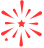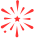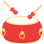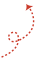# 一文搞懂線性表(順序表、連結串列)### 前言

• 線性表：邏輯結構， 就是對外暴露資料之間的關係，不關心底層如何實現，資料結構的邏輯結構大分類就是線性結構和非線性結構而順序表、連結串列都是一種線性表。

• 順序表、連結串列：物理結構，他是實現一個結構實際實體地址上的結構。比如順序表就是用陣列實現。而連結串列用指標完成主要工作。不同的結構在不同的場景有不同的區別。

### 線性表基本架構

``package LinerList;public interface ListInterface<T> {        void Init(int initsize);//初始化表    int length();    boolean isEmpty();//是否為空    int ElemIndex(T t);//找到編號    T getElem(int index) throws Exception;//根據index獲取資料    void add(int index,T t) throws Exception;//根據index插入資料    void delete(int index) throws Exception;    void add(T t) throws Exception;//尾部插入    void set(int index,T t) throws Exception;    String toString();//轉成String輸出  }``

### 順序表

#### 插入操作

(1)從後(最後一個有資料位)向前到index依次後移一位，騰出index位置的空間

(2)將待插入資料賦值到index位置上，完成插入操作

#### 程式碼實現

``package LinerList;public class seqlist<T> implements ListInterface<T> {    private Object[] date;//陣列存放資料    private int lenth;    public seqlist() {//初始大小預設為10        Init(10);    }    public void Init(int initsize) {//初始化        this.date=new Object[initsize];        lenth=0;            }    public int length() {               return this.lenth;    }    public boolean isEmpty() {//是否為空        if(this.lenth==0)            return true;        return false;    }    /*     * * @param t        * 返回相等結果，為-1為false     */    public int ElemIndex(T t) {        // TODO Auto-generated method stub        for(int i=0;i<date.length;i++)        {            if(date[i].equals(t))            {                return i;            }        }        return -1;    }    /*     *獲得第幾個元素     */    public T getElem(int index) throws Exception {        // TODO Auto-generated method stub        if(index<0||index>lenth-1)            throw new Exception("數值越界");        return (T) date[index];    }    public void add(T t) throws Exception {//尾部插入         add(lenth,t);    }    /*     *根據編號插入     */    public void add(int index, T t) throws Exception {        if(index<0||index>lenth)            throw new Exception("數值越界");        if (lenth==date.length)//擴容        {            Object newdate[]= new Object[lenth*2];            for(int i=0;i<lenth;i++)            {                newdate[i]=date[i];            }            date=newdate;        }        for(int i=lenth-1;i>=index;i--)//後面元素後移動        {            date[i+1]=date[i];        }        date[index]=t;//插入元素        lenth++;//順序表長度+1    }    public void delete(int index) throws Exception {        if(index<0||index>lenth-1)            throw new Exception("數值越界");        for(int i=index;i<lenth;i++)//index之後元素前移動        {            date[i]=date[i+1];        }        lenth--;//長度-1      }    @Override    public void set(int index, T t) throws Exception {        if(index<0||index>lenth-1)            throw new Exception("數值越界");        date[index]=t;    }    public String  toString() {        String vaString="";        for(int i=0;i<lenth;i++)        {            vaString+=date[i].toString()+" ";        }        return vaString;    }}``

### 連結串列

#### 基本結構

``class node<T>{    T data;//結點的結果    node next;//下一個連線的結點    public node(){}    public node(T data)    {        this.data=data;    }    public node(T data, node next) {        this.data = data;        this.next = next;    } }``

#### 插入操作

1. `node.next=pre.next`，將插入結點後面先與連結串列對應部分聯絡起來。此時node.next和pre.next一致。

2. `pre.next=node` 將node結點插入到連結串列中。

#### 程式碼實現

``package LinerList;class node<T>{    T data;//結點的結果    node next;//下一個連線的結點    public node(){}    public node(T data)    {        this.data=data;    }    public node(T data, node next) {        this.data = data;        this.next = next;    }}public class Linkedlist<T> implements ListInterface<T>{    node head;    private int length;    public Linkedlist() {        head=new node();        length=0;    }    public void Init(int initsize) {        head.next=null;    }    public int length() {        return this.length;    }    public boolean isEmpty() {        if(length==0)return true;        else return false;    }    /*     * 獲取元素編號     */    public int ElemIndex(T t) {        node team=head.next;        int index=0;        while(team.next!=null)        {            if(team.data.equals(t))            {                return index;            }            index++;            team=team.next;        }        return -1;//如果找不到    }    @Override    public T getElem(int index) throws Exception {        node team=head.next;        if(index<0||index>length-1)        {            throw new Exception("數值越界");        }        for(int i=0;i<index;i++)        {            team=team.next;        }        return (T) team.data;    }    public void add(T t) throws Exception {        add(length,t);    }    //帶頭結點的插入，第一個和最後一個一樣操作    public void add(int index, T value) throws Exception {        if(index<0||index>length)        {            throw new Exception("數值越界");        }        node<T> team=head;//team 找到當前位置node        for(int i=0;i<index;i++)        {             team=team.next;        }        node<T>node =new node(value);//新建一個node        node.next=team.next;//指向index前位置的下一個指標        team.next=node;//自己變成index位置            length++;    }    @Override    public void delete(int index) throws Exception {        if(index<0||index>length-1)        {            throw new Exception("數值越界");        }        node<T> team=head;//team 找到當前位置node        for(int i=0;i<index;i++)//標記team 前一個結點        {             team=team.next;        }        //team.next結點就是我們要刪除的結點        team.next=team.next.next;        length--;    }    @Override    public void set(int index, T t) throws Exception {        // TODO Auto-generated method stub        if(index<0||index>length-1)        {            throw new Exception("數值越界");        }        node<T> team=head;//team 找到當前位置node        for(int i=0;i<index;i++)        {             team=team.next;        }        team.data=t;//將數值賦值，其他不變    }    public String toString() {        String va="";        node team=head.next;        while(team!=null)        {            va+=team.data+" ";            team=team.next;        }        return va;    }}``

## 推薦閱讀

跳錶 | 會跳的連結串列原來這麼diao
資料結構與演算法之基本概念
「乾貨總結」程式設計師必知必會的十大排序演算法
花5分鐘看這篇之前,你才發現你不懂RESTful
「五大常用演算法」一文圖解分治演算法和思想「其他文章」